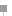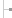Get started Bring yourself up to speed with our introductory content.

# Lotus Notes Formula language general syntax rules

## Here are the general syntax rules for Formula language for Lotus Notes.

The following is tip #5 from "A Formula language for Lotus Notes introduction -- 7 tips in 7 minutes," excerpted from Chapter 5 of the book Lotus Notes Developer's Toolbox , published by IBM Publishing.

Formula language general syntax rules

The Formula Language syntax rules are quite simple. All formulas must adhere to the following five rules.

Rule 1:
A semicolon must separate all formulas that contain more than one statement. It's important to understand that multiple statements can reside on a single line, provided that a semicolon delineates each statement. For example, the following are valid multi-statement formulas.

Example 1

```DEFAULT status := "New Request"; status
```

Example 2

```DEFAULT status := "New Request";
status
```

Rule 2:
At least one space must follow all reserved keywords. Otherwise, any number of spaces --from none to many -- may be used to separate operators, punctuation, and values. For example, the following are valid and equivalent statements (with regard to spacing):

```output := "Today's date is: " + @Today;
output:="Today's date is: " + @Today;
FIELD output:= "Today's date is: " + @Today;
```

Rule 3:
Two values, variables, or strings must be separated by at least one operator. For example, the following are valid statements. In the first example, the plus sign is used to sum the two numbers and divide the result by 2. In the second example, the plus sign is used to concatenate two values together. Finally, the third example shows three @Functions separated by the plus operator.

```output := (100 + 200)/2;
output := "The sum of the two numbers is: " + total;
output := @[email protected][email protected];
```

Rule 4:
You must use the := assignment operator to assign a value to a variable, whereas the equal sign = is used to compare two values when used in a conditional statement (such as the @IF, @WHILE, or @FOR functions).

```myVariable := "This is a valid assignment";
```

Rule 5:
All formulas must contain a main expression that either produces a value or performs an action. For example, the following are valid main expressions. The following returns today's date.

```@Today
```

The following checks if the value stored in the result variable is equal to one. If the condition is true, then the formula returns the text string "Yes". Otherwise, the formula is set to a text string "No."

```@If ( result = 1; "Yes"; "No")
```A Formula language for Lotus Notes introductionHome: IntroductionTip 1: What is Lotus Formula Language?Tip 2: Working with variables in Formula language for Lotus NotesTip 3: Lotus Notes Formula language keywordsTip 4: Working with operators in Formula language for Lotus NotesTip 5: Lotus Notes Formula language general syntax rulesTip 6: What are Lotus Notes Formula language functions and commands?Tip 7: Working with text strings in Formula language for Lotus NotesThis chapter excerpt from Lotus Notes Developer's Toolbox, by Mark Elliott, is printed with permission from IBM Publishing, Copyright 2006. Click here for the chapter download or purchase the book here.

#### Dig Deeper on Lotus Notes 5

• Favorite iSeries cheat sheets

Here you'll find a collection of valuable cheat sheets gathered from across the iSeries/Search400.com community. These cheat ...

Close